﻿ Energy Consumption and Economic Growth Nexus: Empirical Evidence from Tunisia

### Energy Consumption and Economic Growth Nexus: Empirical Evidence from Tunisia

Kais Saidi, Sami HammamiOPEN ACCESSPEER-REVIEWED

## Energy Consumption and Economic Growth Nexus: Empirical Evidence from Tunisia

Kais Saidi1,, Sami Hammami1

1Faculty of Economics and Management, University of Sfax, Street of Airport, LP 1088, Sfax 3018, Tunisia

### Abstract

This article examines the two-way linkages between energy consumption and economic growth using data from Tunisia over the period 1974-2011. This research tests this interrelationship between variables using the Johansen cointegration technique. Our empirical results show that there exists bidirectional causal relationship between energy consumption and economic growth in the long-run. The study suggests that energy policies should recognize the differences in the nexus between energy consumption and economic growth in order to maintain sustainable economic growth in Tunisia.

### At a glance: Figures

1
Prev Next

• Saidi, Kais, and Sami Hammami. "Energy Consumption and Economic Growth Nexus: Empirical Evidence from Tunisia." American Journal of Energy Research 2.4 (2014): 81-89.
• Saidi, K. , & Hammami, S. (2014). Energy Consumption and Economic Growth Nexus: Empirical Evidence from Tunisia. American Journal of Energy Research, 2(4), 81-89.
• Saidi, Kais, and Sami Hammami. "Energy Consumption and Economic Growth Nexus: Empirical Evidence from Tunisia." American Journal of Energy Research 2, no. 4 (2014): 81-89.

 Import into BibTeX Import into EndNote Import into RefMan Import into RefWorks

### 1. Introduction

Since the oil shocks of the 1970s, the energy efficiency is a major concern for all countries of the world, and a growing interest in the study of the relationship between energy consumption and GDP growth. Indeed the level of economic and social development of a country is often linked to the level of energy consumption per capita. Energy plays an important role in the economic growth of both developed and developing countries. The growth hypothesis suggests that energy consumption is an indispensable component in growth, directly or indirectly as a complement to capital and labour as an input in the production process (Mulegeta et al. 2010). Since production and consumption activities involve energy as an essential factor inputs, the relationship between energy consumption and economic growth has been a subject of greater inquiry as energy is considered to be one of the important driving force of economic growth in all economies (Abdulnasser and Manuchehr, 2005). The question as to whether energy consumption has positive, negative or neutral impact on economic activities has motivated the interest of economists and policy analysts hence the need to find out the impact and direction of causality between energy consumption and economic growth (Eddine, 2009).

In literature, the nexus between energy and economic growth has attracted attention of researchers in different countries for a long time. This nexus suggests that higher economic growth requires more energy consumption and more efficient energy use needs a higher level of economic growth. Since the pioneer work of Kraft and Kraft (1978), Granger causality test approach has become a popular tool for studying the relationship between economic growth and energy consumption in different countries, e.g. Stern (1993), Altinay and Karagol (2004), Omotor (2008) and Olusegun (2008), Belloumi (2009), Pao (2009), Odularu and Okonkwo (2009), and Ghosh (2010). However, Altinay and Karagol (2004) investigated the causal relationship between electricity consumption and real GDP in Turkey over the period of 1950–2000. They showed that both used tests have yielded a strong evidence for unidirectional causality running from the electricity consumption to income. This implies that the supply of electricity is vitally important to meet the growing electricity consumption, and hence to sustain economic growth in Turkey. Omotor (2008) and Olusegun (2008) investigated the causality and long run relationship between energy consumption and economic growth, the work of Olusegun (2008) is particularly noteworthy as it is one of the first to apply the ARDL bounds test approach to co-integration. Belloumi (2009) has used a VECM Model and showed that, in Tunisia, there is a causal relationship between energy consumption and income over the period of 1971-2004. Odularu and Okonkwo (2009) their study is limited to the long run relationship between the energy consumption and economic growth. Furthermore, studies by However, the author did not consider coal consumption as one of his respective independent variables. Therefore, this study intends to fill this gap in the literature.

As we can see, the result of the above studies on the relationship between energy consumption and GDP differ from country to another and vary depending to the used methodology (See Table 1). In fact, our paper investigates the two-way between energy consumption and economic growth in Tunisia over the period 1990–2011 using the Johansen co-integration and Granger causality techniques.

#### Table 1. Summary of empirical studies on energy consumption–growth nexus for country-specific studies

Therefore, the major objective of this study is to empirically examine the linkages between the impacts of energy consumption on economic growth in Tunisia. In order to achieve this objective, the paper is organized into five sections including this introduction. The rest of the paper is organized as follows: Section 2 briefly reviews the related literature. Section 3 outlines the econometric modeling approach and describes the data used. Section 4 reports and discussed the empirical results. Section 5 concludes the article and offers some policy implications.

### 2. Energy Consumption and Growth Nexus

Historically, in the literature concerning the scope of our study, there are four generations of models (Mehara, 2007). Indeed, the first generation is based on the traditional VAR model Sims and testing classic Granger causality, it assumes that all macroeconomic series are stationary (Kraft and Kraft, 1978; Akarca and Long, 1980, Yu and Hwang, 1984, Yu and Choi, 1985). The second and third generation augured non -stationary macroeconomic series. They used cointegration as the most appropriate technique. For the second generation, once tested the degree of cointegration between the two series, it estimates the error correction model. Finally, it tests the causal relationship (Yang, 2000, Butt, 2001). In addition, the third generation uses multivariate cointegration approach based on the method of maximum likelihood (Masih and Masih, 1996). The fourth generation of the test procedures used in unit root and cointegration based on panel data (Al- Iriani, 2006, Lee, 2007, 2008, Mahadevan and Asafu - Adaye, 2007).

From a study of Kraft and Kraft (1978), the analysis of the link between energy consumption and economic growth has been studied extensively over the past three decades. However, the evidence is still controversial; the literature of the energy economy has carefully considered the nature of the causal relationship between energy consumption and economic growth. However, there is no consensus on the direction of causality; the findings of empirical studies are divergent. In a study of two countries Ebohon (1996) used to test Granger causality and showed that there is a causal relationship between energy and economic growth in Nigeria and Tanzania.

Asafu-Adjay (2000) in examining the causal relationship between energy consumption, energy prices and economic growth in India, Indonesia, the Philippines and Thailand, uses cointegration techniques modeling error correction. It shows that there is a unidirectional causal relationship between the two series in India and Indonesia. While, a bidirectional causality from energy consumption to income for Thailand and Philippines. However, evidence of two-way relationship is established in the study of Yang (2000) on the Chinese province and Taiwan.

Also, the study Soytas and Sary (2003) for G7 countries and some emerging markets has led to varying conclusions. They suggest the presence of a bi-directional causality in Argentina, unidirectional causality from GDP to energy consumption in Italy and Korea and a unidirectional causality from energy consumption to GDP in Turkey, France, Germany and Japan. Application of cointegration techniques and vector error correction on data from Malawi for the period 1970 to 1999, Jombe (2004) found bidirectional causality between electricity consumption and economic growth, but a unidirectional causality running from GDP power consumption. The studies of Morimoto and Hope (2004) and Wolde- Rufael (2004) in Siri Lanka and Shanghai have shown the existence of a unidirectional causality from energy consumption to GDP. Some other studies have yielded conflicting findings, such as the Oh and Lee (2004) in Korea study indicate a bidirectional causality relationship in the long term and a unidirectional causality from energy consumption to GDP in the short term. Similarly, Wolde- Rufael (2005) also found contradictory and a unidirectional relationship of energy consumption to GDP for African countries results.

In the case of Malaysia, Ang (2008) found that pollution and energy use were positively related to output in the long-run with strong support for causality running from economic growth to energy consumption, both in the short-run and long-run. Bellouni (2009) apply the Johansen cointegration technique to assess the causal relationship between energy consumption and gross domestic product per capita in Tunisia during the period 1971-2004. These results show a relationship of long-term bidirectional causality between the two series and a unidirectional causality in the short term from the energy to GDP. For the same conclusion, Odhiambo (2009) found that there is a unidirectional causal relationship running from energy consumption to economic growth for Tanzania. In contrast to the findings of Soytas and Sari (2009); Halicioglu (2009) found that there was a bi-directional Granger causality (both in short- and long-run) running between carbon emissions and income in Turkey. In a multivariate causality study for China, Zhang and Cheng (2009) found a unidirectional Granger causality running from GDP to energy consumption, and a unidirectional Granger causality running from energy consumption to carbon emissions in the long run but neither carbon emissions nor energy consumption leads economic growth.

These studies show that the results regarding the causal relationship between energy consumption and economic growth are sometimes conflicting and mixed across different countries when time-series analysis is applied to a single country data set as shown in Table 1.

### 3. Methodology, Model, and Data

3.1. Methodology

The methodology adopted is that of Ambapour and Massampa (2005) using cointegration and error correction model to study the relationship of cause and effect between energy consumption and economic growth. The notion of causality used is specified. It is based on the definition of Granger believes that variable is caused by another when there is information in the past, one that is useful in predicting the other, and who are not already contained in its past. Far from being exhaustive, this definition is an essential step in a statistical study.

3.1.1. Causality in the Case of Cointegrated Variables

So far, we are limited to causal analysis in stationary systems. However, over the past twenty years, many articles note that most macroeconomic series are non-stationary, in particular section of Nelson and Plosser (1982). This implies that before applying any estimation method, a thorough analysis of the properties of the series is essential. The main objective is to identify the possible non-stationary series. This is somehow the step of determining their order.

3.1.1.1. Order of Integration of the Series

A non-stationary series Xt is said to be integrated of order d (Xt ~ I (d)) if, having been differentiated d times, it is stationary. In other words, Xt ~ I (d) if and only if (1-L) Xt ~ I (0). Most macroeconomic time series are integrated of order one, they have a unit root. A unique differentiation enough to make them stationary. The simplest example of variable I (1) is the random walk. The most powerful method to determine the order of integration of a series is based on the unit root tests.

•  Unit root tests

The unit root tests are used to detect the presence of unit root in a series. Among the existing unit root tests, we found the test Augmented Dickey-Fuller. This test is the most widely used because of its simplicity. It involves testing the null hypothesis H0: ρ = 1 against the alternative hypothesis H1: │ ρ │ <1. It is based on the least squares estimation of the following three models:Model 1: without constant without trend;Model 2: with constant without trend;Model 3: with constant trend with.

Referring to the values Game Café by Fuller in 1976 and Fuller is sequentially into three stages from the model (3) the model (1):

Step 1: Every time you start by testing the significance of the trend of the model (3) by referring to tables of Dickey-Fuller. If the trend is not significant, we move to the model (2), and if the trend is more significant when we test the null hypothesis of unit root in the manner presented above, it appears that the series is non-stationary must differentiate and start the test procedure on the series in first difference. In this case, the testing process stops and you can work directly on the Xt series.

Step 2: where the model is estimated (2), we begin by testing the significance of the constant. If the constant is not significant is happening in model (1) in step 3. If, against the constant is significant, it also tests the null hypothesis of unit root and proceed in the same way as the first step.

Step 3: we directly test the null hypothesis of unit root

•  Cointegration test

This is the next step after the preliminary tests to verify non-stationary series.

According to "Engle and Granger" (1987), two non-stationary series are cointegrated if their linear combination follows an equilibrium path without ever leaving its average long even if they have divergent developments. In other words, there is a stable long-term evolution of these series. The estimated relationship takes the following form:(1)

Where; Yt is the dependent variable; a' is the vector of coefficients of the explanatory variables; Xt represents the vector of explanatory variables; and Zt is the error term. Zt can be written as a linear combination; a'Xt normalized with respect to Yt and can take the following form:(2)

The relation (2) is thus valid only if Xt and Yt are co-integrated, that is to say Zt stationary. Since Zt stationary, Xt and Yt will tend to vary together in time and may suffer momentary deviations, but cannot deviate without limits. Relation (2) is as a long-term relationship or equilibrium measurement and Zt the deviation from the equilibrium value. Engle and Granger (1987), Engle and Yoo (1987) proposed to determine the relationships existing cointegration in a system with a two-step method.

In a first step, we regret the OLS level variables and we see if the residue of this regression is stationary in a second step. That said, for the test of cointegration between integrated processes of order 1 is estimated by OLS static long-run decline in the levels of variables and then the unit root tests are applied to the estimated residue.

The co-integration test used in this study is that of Johansen (1988, 1991). The Johansen procedure port on the rank of matrix P which determines the number of cointegrating vectors. Two statistics are available: the trace test and the test of maximum eigenvector. Trace test is a test report maximum likelihood of calculating the following statistic:(3)

The null hypothesis tested is r ≤ q, which is to say that there are at most r cointegrating vectors. This test amounts to testing the rank of the matrix P, since testing the existence of r cointegrating vectors in a test of the null hypothesis: Rank (P) = r. On the maximum eigenvalue test, the test statistic is given by:(4)

There are three possibilities. First, r = 0 in the case where all variables are non-stationary, but there is no cointegration. Second, r = N (N is the number of variables in the VAR model) where all the variables are stationary. Third, 0 < r < N if it non-stationary linear combinations of variables. The critical values of these statistics were tabulated including Johansen (1988) and Johansen and Jueslieus (1990).

According to the Granger representation theorem, any cointegrated system implies the existence of a mechanism that prevents error variables stray too far from their long-run equilibrium adjustment.

In general, the error correction models are used to model adjustments that lead to a situation of long-term equilibrium. These are actually dynamic models that incorporate both short-term developments and long-term variables. The error correction model is written as follows: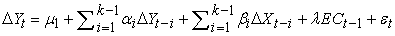(5)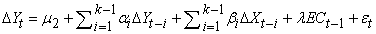(6)

Where εt and εt' are both white noise; ECt-1 is estimated from the cointegration residues delayed by a period, and the coefficients λ and λ' are the respective adjustment speeds. The cointegrating relationship reflects the long-term equilibrium and short-run dynamics of the variables into account fluctuations around the long-term relationship.

•  Causality test

The concept of Granger causality is a theoretical approach to causation which refers not to the theoretical nature of causality (cause and effect), but the predictive nature of the possible cause of the effect. According to Granger, a variable X cause variable Y, if knowledge of the lagged values of the same variable, and lagged values of X that is considered causal variable.

A version of the Granger test directly after the previous representation provides an estimate by the method of least squares the following two equations: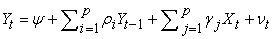(7)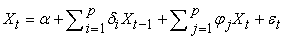(8)

Test assumptions attached to conclude on the direction of causality. Xt and Yt causes in the Granger sense, if the null hypothesis defined above can be rejected in favor of the alternative hypothesis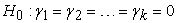;Similarly, Yt causes Xt to Granger. If the null hypothesis defined above, can be rejected in favor of the alternative hypothesis: H0: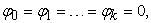H0: au moins un desEngle and Granger (1991) showed that if the variables are integrated, the classical Granger test based on the VAR is no longer appropriate. They recommend making use of the error correction model. In addition, the causality test based on vector model for correcting this error the advantage of providing a causal relationship even if no estimated variable interest offset coefficient is significant. Therefore we rewrite equations (7) and (8) the following manner: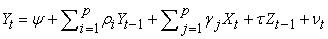(9)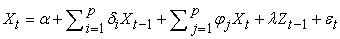(10)

Using the vector error-correction model, Xt does not cause Yt in the Granger sense if γ = τ = 0; Yt does not cause Xt if φ = λ = 0.

3.2. Empirical Model

The model we tested expressed a hypothesis which is the link between energy consumption, the income represented by economic activity and the relative price of energy is introduced as an additional variable GDP = f (EC, EP). The relationships built through this basic proposal are:With GDPt: The level of income, ECt: energy consumption and EPt: The energy prices.

3.3. Data Source and Descriptive Statistic

About our field of study, in most studies, it is often difficult to collect statistics for a country. This finding is particularly true when it comes to statistics on African countries. These are either non-existent or published occasionally so that it is often with limited data.

In many studies concerning the subject matter hereof, the terms of economic growth, energy consumption and energy prices are not clearly defined. A number of variables are often used to represent them. As a proxy for economic growth, is most often used is the GDP, GNP, and in some cases, national income and industrial production. Regarding energy consumption, it is considered to be the total consumption or an aggregate index, weighted by the different energy sources. So far the price of energy can be used as a proxy of the price of total energy, or the index of consumer prices.

To study the relationship between energy consumption and economic growth, annual data is extracted from World Bank’s World Development Indicators 2012 and the National Institute of Statistics 2009 for the period 1974–2011. This paper uses annual time series data which include the real GDP per capita (constant 2000 US\$), energy consumption (kg of oil equivalent per capita), and the price of energy (the index of consumer prices) for Tunisia.

The descriptive statistics, the mean value, the standard deviation, the Min and Max of different variables for individuals and also for the panel are given below in Table 2. This table provides a statistical summary associated with the actual values of the used variables for Tunisia. The highest means of GDP per capita (23.265), energy consumption (6.093), and price of energy (3.979). The highest standard deviation of GDP per capita (0.9643), energy consumption (0.666), and price of energy (0.665).

#### Table 2. Descriptive statistics of variables

Figure 1. Evolution series LGDP, LEC, LEP

### 4. Empirical Findings and Discussion

The causal relationship between energy consumption and GDP has been studied extensively over the past three decades. Large literatures reviewed in this area are evidence of a unidirectional, bidirectional, or no causality in the countries surveyed. However, in our Tunisian case, we will see the result of causality between energy consumption and GDP by applying the Granger causality test.

4.1. Unit Root Test

The results of the unit root test are presented in Table 3. The delays were optimized by both Akaike and Schwarz criteria. The above table gives the results of unit root tests that we performed.

Table 3 provides information on the presence of a unit root level for each of the cate series and no unit root dice the first differentiation. So the three variables (GDP, EN, and PE) are stationary in first differences. They are then integrated of order 1 [I (1)]. The null hypothesis of unit root is accepted at 5% level. It is therefore possible that they are cointegrated.

4.2. Johansen Co-integration Test

Given the results of unit root tests, cointegration tests were conducted to demonstrate the existence of a stable long-term relationship between the level of GDP, energy consumption (EC) and the price of energy (PE).

A preliminary step is to determine the number of delays in writing the VAR model using information criteria to be AIC and Schwarz. The results provided by these two criteria are shown in the Table 4:

According to this table the number of lags is optimized K = 2. Remember that the different sub-models of the tested model are as follows:

Model 1: There is no constant and linear trend in the VAR and the cointegrating relationship does not include more constant and linear trend.

Model 2: There is no constant and linear trend in the VAR, but the cointegrating relationship includes a constant [no linear trend].

Model 3: There are constant [no linear trend] in the VAR and the cointegrating relationship includes a constant [no linear trend].

Model 4: There are constant [no linear trend] in the VAR model, the cointegrating relationship includes a constant and a linear trend.

Model 5: There are constant and trend in the VAR and the cointegrating relationship includes a constant and a linear trend.

In testing these sub-models with a delay k = 2, we find that the optimized model is the one (or constant or trend), r = 1, k = 2. The Johansen test will be conducted from this model r = 1 with a delay k = 2. The test results are shown in the Table 5.

This table shows that the null hypothesis (where more) r = 0 [for the test track] or exactly r = 0 [for the maximum eigenvalue test] is rejected at the 5% threshold 1 %. This stems from the values calculated from these two statistics [37, 30 for the Trace test and 27.52 for the maximum eigenvalue test]. For cons, the null hypothesis r ≤ 1 [for testing trace] or r = 1 [for the maximum eigenvalue test] cannot be rejected at the 5% and 1% for the two test statistics Johansen are below the critical values associated with them even for the null hypothesis r ≤ 2 [for testing trace] or r = 2 [for the maximum Eigen value test]. Both Johansen co-integration tests thus confirm the existence of one cointegrating relationship.

The standard relationship:(11)

The variables in parentheses represent the Student statistic. In other words, a 1% increase in energy consumption would lead to a long-term increase of 8.3% of GDP. In contrast, a 1% increase in energy prices would reduce GDP by 7.1%.

4.3. Estimation of the Model Error-correction

As a reminder, the representation theorem of Engle and Granger shows that non-stationary series especially those with a unit root, must be represented as a model for error correction if they are cointegrated, that if there is a stationary linear combination there between. The estimation of the vector error-correction model requires the determination of the long-term relationship below:(12)

The variables in parentheses represent the Student statistic.

This co-integrating relationship shows that the EC variable, which measures the energy consumption, has a positive relationship with economic growth (LPIB). In the long term, if the energy consumption by 1%, while economic growth follows the same trend with a percentage of 9% to ensure this cointegrating relationship, which shows the decoupling of economic growth from the energy consumption. However, when the energy price increases of 1% economic growth falls by 10%. The estimated error correction model is given in the Table 6.

#### Table 6. Result of test model error correction

The first line contains the endogenous variables and the first column of the exogenous variables, the error correction term, the coefficient of determination and the Fisher statistic. The three estimated equations can be written: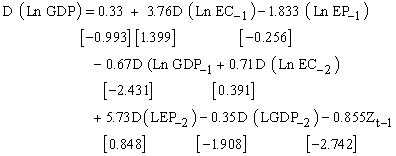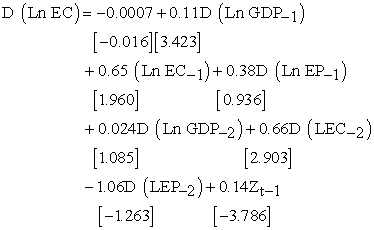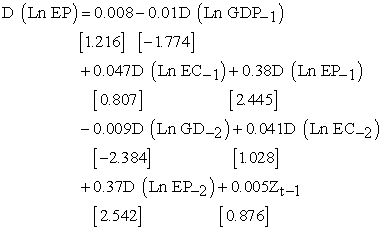The quality of the estimation of this model is good under the Fisher statistic and the coefficient of determination.

In reviewing this representation, we note that the error correction term is negative and significant in the relationship relative to GDP (first equation), thus confirming the existence of a long-term relationship between energy consumption and growth. The correction model can be validated in this case. So the equation that represents the short-term adjustments, (equation relative to GDP) is the following: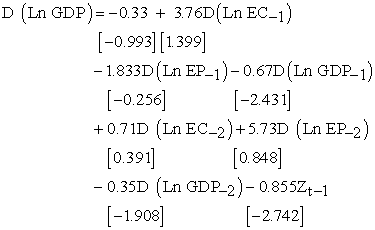This correction is made by the restoring force of 85.5% remains significantly above that it is justified by the Student statistic (-2.74). Therefore when there is deviation, the system automatically comes to fix.

4.4. Granger Causality Test

Most studies regarding our scope were primarily designed to answer the question posed by Masih and Masih (1989): "Does economic growth precedence take over energy use, energy use or can be a stimulus Itself for economic growth through the indirect channels of effective aggregate demand and human capital, Improved efficiency and technological progress? « In other words:

•  GDP is it because of the energy consumption: LEC = f (LGDP)?

•  Energy consumption is it because GDP = f (LEC)?

In both cases, we added two other cases often encountered:

•  The existence of bidirectional causality between GDP and energy consumption.

•  The two variables are independent.

This causal relationship examined by using Granger is based on the vector error correction model. The results of this test are shown in the Table 7.

#### Table 7. Results of Granger causality test

From this Table 7, six hypotheses were tested simultaneously, namely the causality between the three variables taken in pairs. We tested the hypothesis and to know if the power consumption does not cause economic growth and vice versa. The same assumptions have been taken between the relative energy prices and economic growth and between the relative price of energy and energy consumption.

We note that 5% threshold; the Granger test suggests, on the one hand, a two-way causal link between energy consumption and economic growth, as well as between energy consumption and price on energy. Secondly, a unidirectional relationship between the relative energy prices and economic growth. In other words, it is energy that causes economic growth and not the reverse the price.

Our result for Tunisia is compatible with the flattering idea that energy consumption has a causal effect on economic growth. It was also confirmed the findings of Stern (1993, 2000), Yang (2000) and Asafu-Adjaye (2000) obtained similar results for other countries. In addition, he rejects the neo-classical hypothesis which says that energy to a neutral effect on economic growth.

### 5. Conclusion and Policy Implications

This paper has attempted to analyze empirically the interaction between energy consumption and economic growth for Tunisia. This study has demonstrated that the causal relationship between the two variables in Tunisia is mixed or conflicting. Our empirical results using the standard Granger causality test reveal a bidirectional causal relationship between energy consumption and growth. However, based on the Johansen test, our results show also a bidirectional causality relationship between GDP and energy consumption in Tunisia. This significantly rejects the neo-classical assumption of the neutrality of the effect of energy on economic growth. As a result, energy is a limiting factor for growth in Tunisia. In fact, any shock in energy demand can affect growth. These results are consistent with the findings of Cheng (1999) and Asafu Adjaye (2000). These imply that energy is a determinant factor of the GDP growth in Tunisia, and, therefore, a high-level of economic growth leads to a high level of energy demand and vice versa. As such, it is important to take into account their possible negative effects on economic growth in establishing energy conservation policies. Indeed, the direction of causality can help policy makers to make the most appropriate decisions on climate policy: for example, evidence of unidirectional causality from income to energy consumption could assume full compatibility between political energy conservations and policies for economic growth, since the former can be continued without limit seconds. Therefore, the Tunisian government should encourage research and development on technological innovation for energy savings. In doing so, we could simultaneously reduce environmental degrading and also enhance economic development in the Tunisian economy. In addition to that, alternative energies such as solar power and wind power should be considered because these alternative energies are more environmental friendly compare to fossil fuel.

### References

  Akarca, A.T., Long, T.V., 1980. On the relationship between energy and GNP: a re-examination. Journal of Energy Development 5, 326-331.In article  Al-Iriani, M.A; 2006. Energy-GDP relationship revisited: An example from GCC countries using panel causality. Energy Policy 34, 3342-3350.In article CrossRef  Altinay, G., Karagol, E., 2004. Structural break, unit root, and the causality between energy consumption and GDP in Turkey. Energy Economics 26, 985-994In article CrossRef  Altinay, G., Karagol, E., 2004. Structural break, unit root, and the causality between energy consumption and GDP in Turkey. Energy Economics 26 (6), 985-994.In article CrossRef  Ang, J., 2008. Economic development, pollutant emissions and energy consumption in Malaysia. J. Policy Model. 30, 271-278.In article CrossRef  Aqeel, A., Butt, M.S., 2001. The relationship between energy consumption and economic growth in Pakistan. Asia-Pacific Development Journal 8, 101-109.In article  Asafu-Adjaye, J., 2000. The relationship between energy consumption, energy prices and economic growth: Time series evidence from Asian developing countries. Energy Economics 22, 615-625.In article CrossRef  Belloumi, M., 2009. Energy consumption and GDP in Tunisia: Cointegration and causality analysis. Energy policy 37, 2745-2753.In article CrossRef  Chandran, V.G.R., Sharma, S., Madhavan, K., 2010. Electricity consumption-growth nexus: the case of Malaysia. Energy Policy 38, 606-612.In article CrossRef  Cheng, B.S., 1999. Causality between energy consumption and economic growth in India: an application of cointegration and error-correction modeling. Indian Economic Review xxxiv, 39-49.In article  Ebohon, O.J., 1996. Energy, economic growth and causality in developing countries. Energy Policy 24, 447-453.In article CrossRef  Erdal, G., Erdal, H., Esengun, K., 2008. The causality between energy consumption and economic growth inTurkey. Energy Policy36(10), 3838-3842.In article CrossRef  Ghosh, S., 2009. Electricity supply, employment and real GDP in India: evidence from cointegration and Granger-causality tests. Energy Policy 37 (8), 2926-2929.In article CrossRef  Granger C.W.J., 1991. Developments in the study of cointegrated economic variables: In Long-run economic relation/Readings in cointegration (Eds.) Engle RF, Granger C.W.J., Oxford University Press, Oxford, 65-80.In article  Halicioglu, F., 2009. An econometric study of CO2 emissions, energy consumption, income and foreign trade in Turkey. Energy Policy 37, 1156-1164.In article CrossRef  Jamil, F., Ahmad, E., 2010. The relationship between electricity consumption, electricity prices and GDP in Pakistan. Energy Policy 38, 6016-6025.In article CrossRef  Johansen, S., 1988. Statistical analysis of cointegrating vectors. Journal of Economic Dynamics and control 12, 231-254.In article CrossRef  Johansen. S., 1991. Estimation and Hypothesis Testing of Cointegration Vectors in Gaussian Vector Autoregressive Models. Econometrica 59, 1551-1580.In article CrossRef  Jumbe, C.B.L., 2004. Cointegration and causality between electricity consumption and GDP: Empirical evidence from Malawi. Energy Economics 26, 61-68.In article CrossRef  Jumbe, C.B.L., 2004. Cointegration and causality between electricity consumption and GDP: Empirical evidence from Malawi. Energy Economics 26, 61-68.In article CrossRef  Kraft, J; Kraft, A., 1978. On the Relationship between Energy and GNP. Journal of Energy and Development 3, 401-403.In article  Lean, H.H., Smyth, R., 2010. On the dynamics of aggregate output, electricity consumption and exports in Malaysia: evidence from multivariate Granger causality tests. Applied Energy 87, 1963-1971.In article CrossRef  Lee, C.C., Chang, C.P., 2008. Energy consumption and economic growth in Asian economies: a more comprehensive analysis using panel data. Resource and Energy Economics 30 (1), 50-65.In article CrossRef  Lee, C.C., Chang, C.P., Chen, P.F., 2008. Energy–income causality in OECD countries revisited: the key role of capital stock. Energy Economics 30, 2359-2373.In article CrossRef  Mahadevan, R., Asafu-Adjaye, J., 2007. Energy consumption, economic growth and prices: reassessment using panel VECM for developed and developing countries. Energy Policy 35, 2481-2490.In article CrossRef  Masih, A. M. M; Masih, R., 1996. Energy consumption, real income and temporal causality: Results from a multi-country study based on cointegration and error-correction modelling techniques. Energy Economics 18, 165-183.In article CrossRef  Mehrara, Mohsen., 2007. Energy consumption and economic growth: The case of oil exporting countries. Energy Policy, Elsevier 35, 2939-2945.In article CrossRef  Morimoto, R., Hope, C., 2004. The Impact of Electricity Supply on Economic Growth in Sri Lanka. Energy Economics 26, 77-85.In article CrossRef  Narayan, P.K, Smyth, R., 2008. Energy consumption and real GDP in G7 countries: New evidence from panel cointegration with structural breaks. Energy Economics 30, 2331-2341.In article CrossRef  Odhiambo, N.M., 2009a. Electricity consumption and economic growth in South Africa: a trivariate causality test. Energy Economics 31, 635-640.In article CrossRef  Odhiambo, N.M., 2009b. Energy consumption and economic growth nexus in Tanzania: an ARDL bounds testing approach. Energy Policy 37, 617-622.In article CrossRef  Odularo, G.O. and Okonkwo, C., 2009. Does Energy Consumption Contribute to Economic Performance? Empirical Evidence from Nigeria. Journal of Economics and International Finance 1, 044-058.In article  Oh, W., & Lee, K., 2004. Causal relationship between energy consumption and GDP revisited: The case of Korea 1970–1999. Energy Economics 26, 51-59.In article CrossRef  Olusegun, O.A., 2008. Energy consumption and Economic Growth in Nigeria: A Bounds Testing Co-inntegrative Approach. Journal of Economic Theory 2, 118-123.In article  Omotor, D.G. (2008). “Causality between Energy Consumption and Economic Growth in Nigeria.” Pakistan Journal of Social Sciences, Vol. 5 (8), pp. 827-835.In article  Ouedraogo, M., 2010. Electricity consumption and economic growth in Burkina Faso: a cointegration analysis. Energy Economics 3, 524-531.In article CrossRef  Soytas, U., Sari, R., 2003. Energy Consumption and GDP: Causality Relationship in G-7 Countries and Emerging Markets. Energy Economics 25, 33-37.In article CrossRef  Soytas, U., Sari, R., Ewing, B.T., 2007. Energy consumption, income, and carbon emissions in the United States. Ecol. Econ. 62, 482-489.In article CrossRef  Stern, D.I., 1993. Energy and economic growth in the USA. Energy Economics 15, 137-150.In article CrossRef  Stern, D.I., 2000. Multivariate cointegration analysis of the role of energy in the US macroeconomy. Energy Economics 22, 267-283.In article CrossRef  Tang, C.F., 2008. A re-examination of the relationship between electricity con- sumption and economic growth in Malaysia. Energy Policy 36, 3077-3085.In article CrossRef  Wolde-Rufael, Y., 2004. Disaggregated energy consumption and GDP: The experience of Shangai, 1952-1999. Energy Economics 26, 69-75.In article CrossRef  Wolde-Rufael, Y., 2005. Energy demand and economic growth: The African Experience. Journal of Policy Modeling 27, 891-903.In article CrossRef  Yang, H. Y., 2000. A Note of the Causal Relationship between Energy and GDP in Taiwan. Energy Economics 22, 309-317.In article CrossRef  Yoo, S.H., 2005. Electricity consumption and economic growth: evidence from Korea. Energy Policy 33, 1627-1632.In article CrossRef  Yu, E.S.H., Choi, J.Y., 1985. The causal relationship between energy and GNP: An International Comparison. Journal of Energy Development 10, 249-272.In article  Yu, E.S.H., Hwang, B.K., 1984. The relationship between energy and GNP: further results. Energy Economics. 6, 186-190.In article CrossRef  Zhang, X.-P., Cheng, X.-M., 2009. Energy consumption, carbon emissions, and economic growth in China. Ecol. Econ. 68, 2706-2712.In article CrossRef